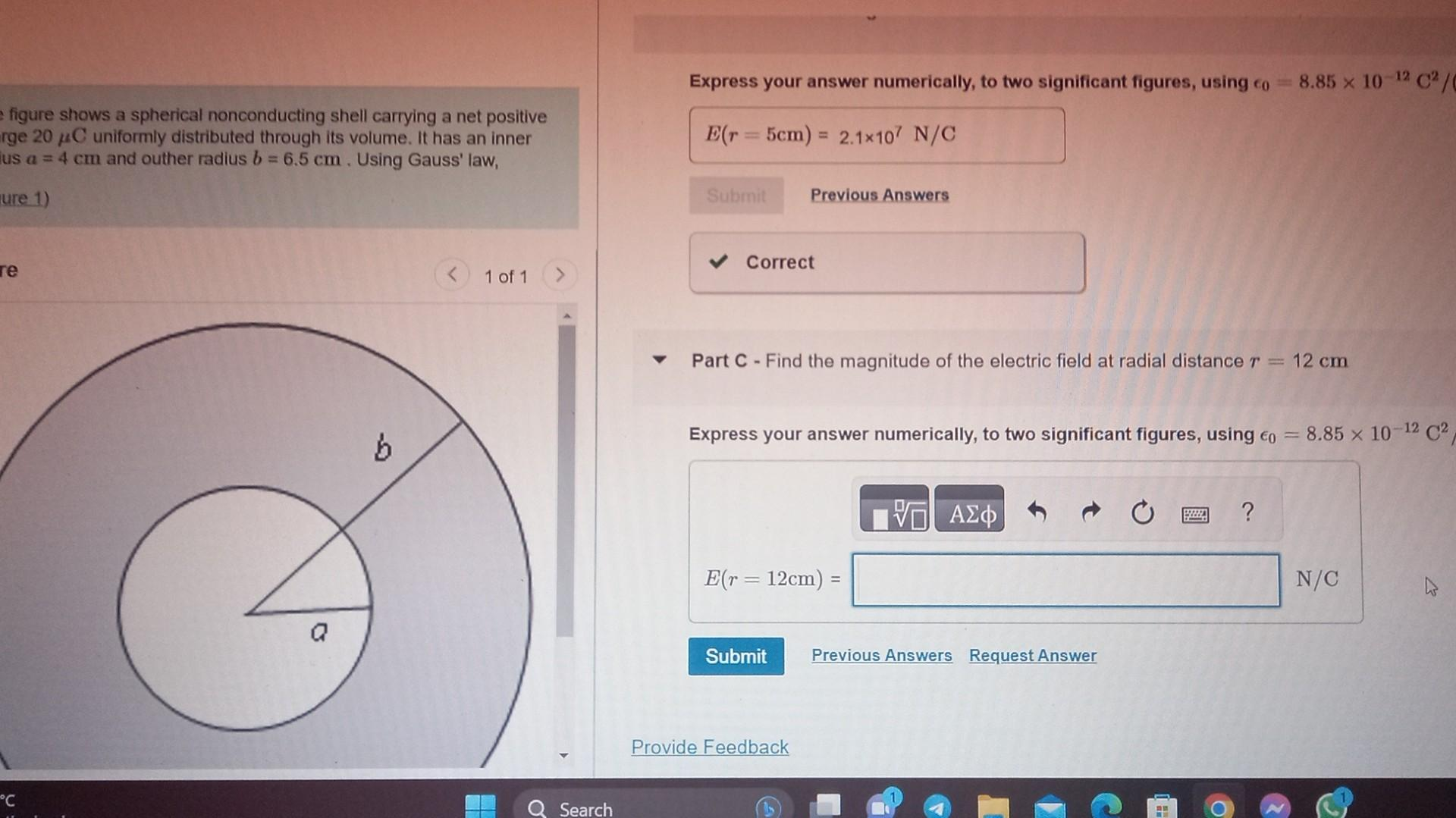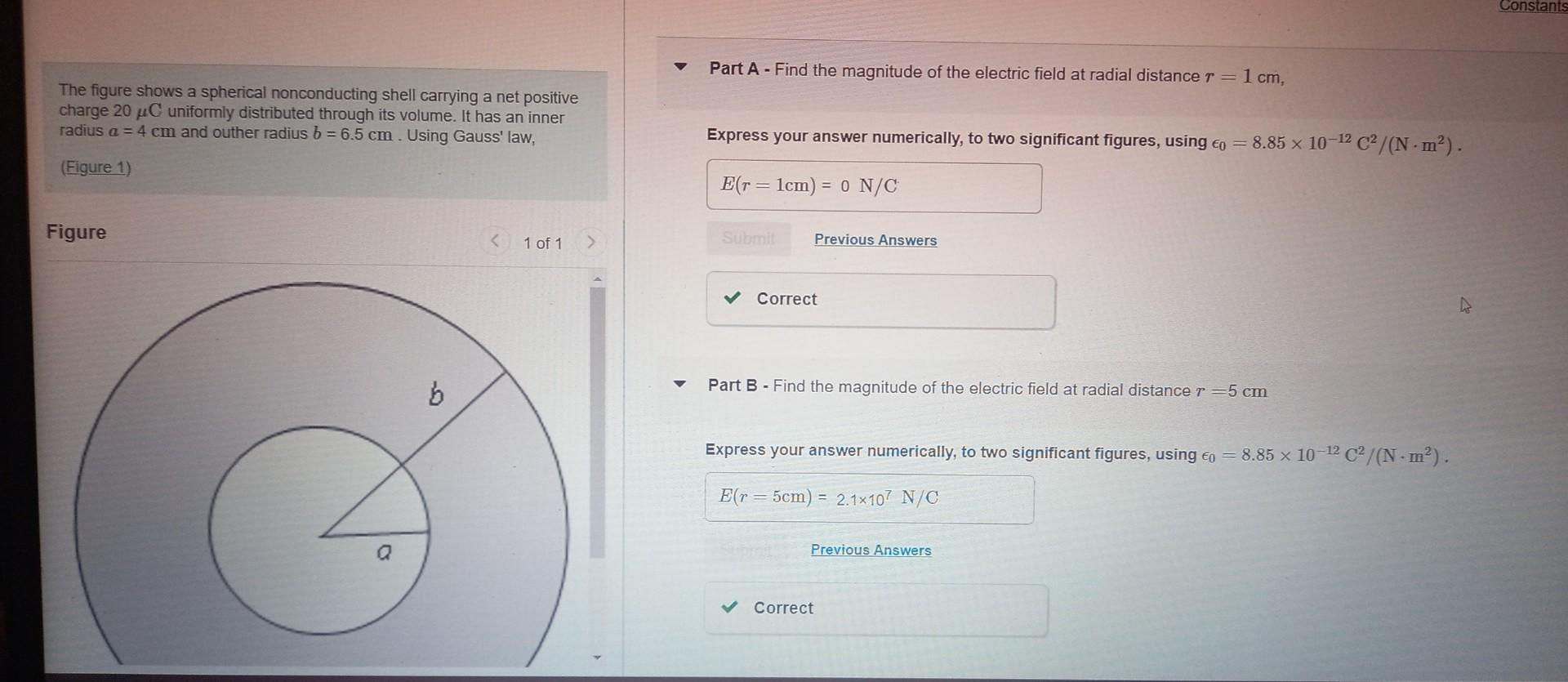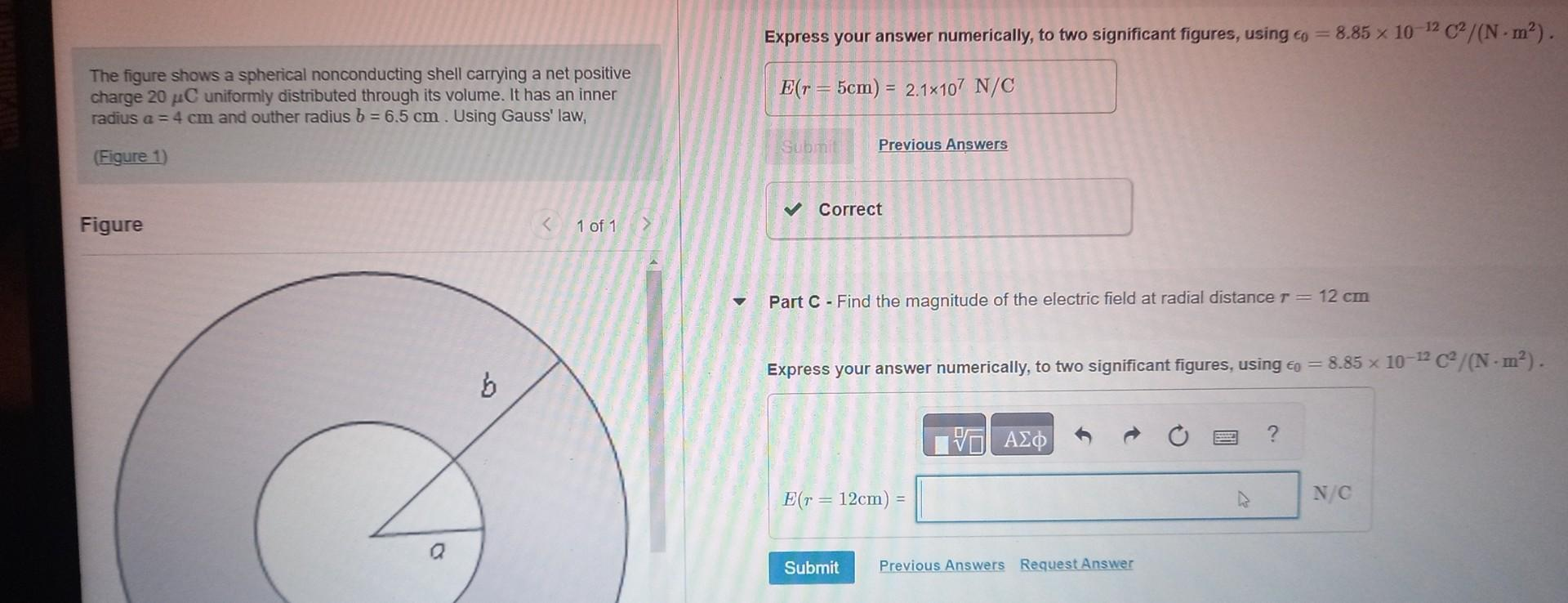# Question the awnser is not 1.25×10^7!!     what is the awnser of part c hint:its not 1.25×10^7 Express your answer numerically, to two significant figures, using $$\epsilon_{0}=8.85 \times 10^{-12} \mathrm{C}^{2} /$$ figure shows a spherical nonconducting shell carrying a net positive rge $$20 \mu \mathrm{C}$$ uniformly distributed through its volume. It has an inner 'us $$a=4 \mathrm{~cm}$$ and outher radius $$b=6.5 \mathrm{~cm}$$. Using Gauss' law, ure 1) Part C - Find the magnitude of the electric field at radial distance $$r=12 \mathrm{~cm}$$ Express your answer numerically, to two significant figures, using $$\epsilon_{0}=8.85 \times 10^{-12} \mathrm{C}^{2}$$, Part A - Find the magnitude of the electric field at radial distance $$r=1 \mathrm{~cm}$$, The figure shows a spherical nonconducting shell carrying a net positive charge $$20 \mu \mathrm{C}$$ uniformly distributed through its volume. It has an inner radius $$a=4 \mathrm{~cm}$$ and outher radius $$b=6.5 \mathrm{~cm}$$. Using Gauss' law, (Figure 1) Figure Part B - Find the magnitude of the electric field at radial distance $$r=5 \mathrm{~cm}$$ Express your answer numerically, to two significant figures, using $$\epsilon_{0}=8.85 \times 10^{-12} \mathrm{C}^{2} /\left(\mathrm{N} \cdot \mathrm{m}^{2}\right)$$. Express your answer numerically, to two significant figures, using $$\epsilon_{0}=8.85 \times 10^{-12} \mathrm{C}^{2} /\left(\mathrm{N} \cdot \mathrm{m}^{2}\right)$$. The figure shows a spherical nonconducting shell carrying a net positive charge $$20 \mu \mathrm{C}$$ uniformly distributed through its volume. It has an inner radius $$a=4 \mathrm{~cm}$$ and outher radius $$b=6.5 \mathrm{~cm}$$. Using Gauss' law, (Figure 1) Figure 1 of 1 Part C - Find the magnitude of the electric field at radial distance $$r=12 \mathrm{~cm}$$ Express your answer numerically, to two significant figures, using $$\epsilon_{0}=8.85 \times 10^{-12} \mathrm{C}^{2} /\left(\mathrm{N} \cdot \mathrm{m}^{2}\right)$$.the awnser is not 1.25×10^7!!what is the awnser of part c
hint:its not 1.25×10^7

Transcribed Image Text: Express your answer numerically, to two significant figures, using $$\epsilon_{0}=8.85 \times 10^{-12} \mathrm{C}^{2} /$$ figure shows a spherical nonconducting shell carrying a net positive rge $$20 \mu \mathrm{C}$$ uniformly distributed through its volume. It has an inner 'us $$a=4 \mathrm{~cm}$$ and outher radius $$b=6.5 \mathrm{~cm}$$. Using Gauss' law, ure 1) Part C - Find the magnitude of the electric field at radial distance $$r=12 \mathrm{~cm}$$ Express your answer numerically, to two significant figures, using $$\epsilon_{0}=8.85 \times 10^{-12} \mathrm{C}^{2}$$, Part A - Find the magnitude of the electric field at radial distance $$r=1 \mathrm{~cm}$$, The figure shows a spherical nonconducting shell carrying a net positive charge $$20 \mu \mathrm{C}$$ uniformly distributed through its volume. It has an inner radius $$a=4 \mathrm{~cm}$$ and outher radius $$b=6.5 \mathrm{~cm}$$. Using Gauss' law, (Figure 1) Figure Part B - Find the magnitude of the electric field at radial distance $$r=5 \mathrm{~cm}$$ Express your answer numerically, to two significant figures, using $$\epsilon_{0}=8.85 \times 10^{-12} \mathrm{C}^{2} /\left(\mathrm{N} \cdot \mathrm{m}^{2}\right)$$. Express your answer numerically, to two significant figures, using $$\epsilon_{0}=8.85 \times 10^{-12} \mathrm{C}^{2} /\left(\mathrm{N} \cdot \mathrm{m}^{2}\right)$$. The figure shows a spherical nonconducting shell carrying a net positive charge $$20 \mu \mathrm{C}$$ uniformly distributed through its volume. It has an inner radius $$a=4 \mathrm{~cm}$$ and outher radius $$b=6.5 \mathrm{~cm}$$. Using Gauss' law, (Figure 1) Figure 1 of 1 Part C - Find the magnitude of the electric field at radial distance $$r=12 \mathrm{~cm}$$ Express your answer numerically, to two significant figures, using $$\epsilon_{0}=8.85 \times 10^{-12} \mathrm{C}^{2} /\left(\mathrm{N} \cdot \mathrm{m}^{2}\right)$$.
More
Transcribed Image Text: Express your answer numerically, to two significant figures, using $$\epsilon_{0}=8.85 \times 10^{-12} \mathrm{C}^{2} /$$ figure shows a spherical nonconducting shell carrying a net positive rge $$20 \mu \mathrm{C}$$ uniformly distributed through its volume. It has an inner 'us $$a=4 \mathrm{~cm}$$ and outher radius $$b=6.5 \mathrm{~cm}$$. Using Gauss' law, ure 1) Part C - Find the magnitude of the electric field at radial distance $$r=12 \mathrm{~cm}$$ Express your answer numerically, to two significant figures, using $$\epsilon_{0}=8.85 \times 10^{-12} \mathrm{C}^{2}$$, Part A - Find the magnitude of the electric field at radial distance $$r=1 \mathrm{~cm}$$, The figure shows a spherical nonconducting shell carrying a net positive charge $$20 \mu \mathrm{C}$$ uniformly distributed through its volume. It has an inner radius $$a=4 \mathrm{~cm}$$ and outher radius $$b=6.5 \mathrm{~cm}$$. Using Gauss' law, (Figure 1) Figure Part B - Find the magnitude of the electric field at radial distance $$r=5 \mathrm{~cm}$$ Express your answer numerically, to two significant figures, using $$\epsilon_{0}=8.85 \times 10^{-12} \mathrm{C}^{2} /\left(\mathrm{N} \cdot \mathrm{m}^{2}\right)$$. Express your answer numerically, to two significant figures, using $$\epsilon_{0}=8.85 \times 10^{-12} \mathrm{C}^{2} /\left(\mathrm{N} \cdot \mathrm{m}^{2}\right)$$. The figure shows a spherical nonconducting shell carrying a net positive charge $$20 \mu \mathrm{C}$$ uniformly distributed through its volume. It has an inner radius $$a=4 \mathrm{~cm}$$ and outher radius $$b=6.5 \mathrm{~cm}$$. Using Gauss' law, (Figure 1) Figure 1 of 1 Part C - Find the magnitude of the electric field at radial distance $$r=12 \mathrm{~cm}$$ Express your answer numerically, to two significant figures, using $$\epsilon_{0}=8.85 \times 10^{-12} \mathrm{C}^{2} /\left(\mathrm{N} \cdot \mathrm{m}^{2}\right)$$.&#12304;General guidance&#12305;The answer provided below has been developed in a clear step by step manner.Step1/4Explanation:Guass law states that the total electric flux emerging out from a closed surface is equal to the product of sum of all enclosed charges by this surface and the constant $$\mathrm{\frac{{1}}{\epsilon}}$$given,charge in shell is 20ucouter radius = 6.5cminner radius = 4cmExplanation:Please refer to solution in this step.Step2/4Explanation:foe part A there is no enclosed charge so electric field intensity will be zeroby applying gauss law the electric field at r= 1cm will be zeroExplanation:Please refer to solution in this step.Step3/4Explanation:for part B r = 5cm is between inner radius and outer radiusby applying gauss law \( \ ... See the full answer